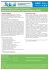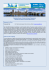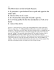# 14-1 Concept-Development Practice Page Satellite Motion

## Transcription

14-1 Concept-Development Practice Page Satellite Motion
```Name
Class
Date
Concept-Development
Practice Page
14-1
Satellite Motion
1. Figure A shows “Newton’s Mountain,” so high that its top is above the drag of the atmosphere. The
cannonball is ﬁred and hits the ground as shown.
a. Draw the path the cannonball might take if it were ﬁred a
little bit faster.
b. Repeat for a still greater speed, but still less than 8 km/s.
c. Draw the orbital path it would take if its speed were
8 km/s.
d. What is the shape of the 8 km/s curve?
e. What would be the shape of the orbital path if the
cannonball were ﬁred at a speed of about 9 km/s?
2. Figure B shows a satellite in circular orbit.
a. At each of the four positions draw a vector that represents the
gravitational force exerted on the satellite.
b. Label the force vectors F.
c. Draw at each position a vector to represent the velocity of the
satellite at that position, and label it v.
d. Are all four F vectors the same length? Why or why not?
e. Are all four v vectors the same length? Why or why not?
f. What is the angle between your F and v vectors?
g. Is there any component of F along v?
h. What does this tell you about the work the force of gravity does on the satellite?
i. Does the KE of the satellite in Figure B remain constant, or does it vary?
j. Does the PE of the satellite remain constant, or does it vary?
CONCEPTUAL PHYSICS
Chapter 14
Satellite Motion
77
3. Figure C shows a satellite in elliptical orbit.
a. Repeat the procedure you used for the circular orbit, drawing vectors F and v for each position,
including proper labeling. Show equal magnitudes with equal lengths, and greater magnitudes
with greater lengths, but don’t bother making the scale accurate.
b. Are your vectors F all the same magnitude?
Why or why not?
c. Are your vectors v all the same magnitude?
Why or why not?
d. Is the angle between vectors F and v everywhere the same,
or does it vary?
e. Are there places where there is a component
of F along v?
g. When there is a component of F along and opposite
to the direction of v, does this increase or decrease
the KE of the satellite?
h. What can you say about the sum KE + PE along the orbit?
CONCEPTUAL PHYSICS
78
Chapter 14
Satellite Motion
f. Is work done on the satellite when there is a component
of F along and in the same direction of v and if so, does this
increase or decrease the KE of the satellite?
```

### CEFD JUN 05 SatMag 3 Quarter page.cdr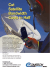### Satellite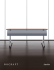### YAMAL-202 49E - Gazprom Space Systems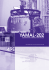### - Gazprom Space Systems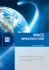### RigNet Case Study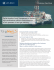### Satellite Weather - Heads Up Technologies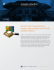### Skycasters Capabilities Statement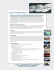### Satellite pictures workshop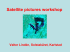### Antenne Bayern Chooses 2wcom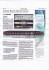### Our Brochure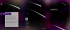### Q1. (a) The diagram shows a satellite moving in a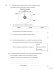### Document 6569566